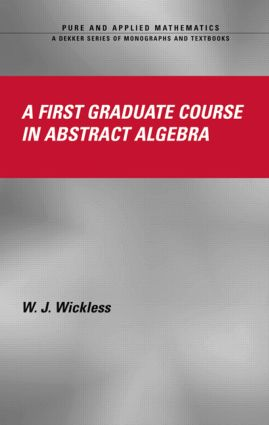# A First Graduate Course in Abstract Algebra

## 1st Edition

CRC Press

252 pages

##### Purchasing Options:\$ = USD
Hardback: 9780824756277
pub: 2004-03-29
\$135.00
x
eBook (VitalSource) : 9781315273228
pub: 2017-11-22
from \$67.50

FREE Standard Shipping!

### Description

Since abstract algebra is so important to the study of advanced mathematics, it is critical that students have a firm grasp of its principles and underlying theories before moving on to further study. To accomplish this, they require a concise, accessible, user-friendly textbook that is both challenging and stimulating. A First Graduate Course in Abstract Algebra is just such a textbook.

Divided into two sections, this book covers both the standard topics (groups, modules, rings, and vector spaces) associated with abstract algebra and more advanced topics such as Galois fields, noncommutative rings, group extensions, and Abelian groups. The author includes review material where needed instead of in a single chapter, giving convenient access with minimal page turning. He also provides ample examples, exercises, and problem sets to reinforce the material. This book illustrates the theory of finitely generated modules over principal ideal domains, discusses tensor products, and demonstrates the development of determinants. It also covers Sylow theory and Jordan canonical form.

A First Graduate Course in Abstract Algebra is ideal for a two-semester course, providing enough examples, problems, and exercises for a deep understanding. Each of the final three chapters is logically independent and can be covered in any order, perfect for a customized syllabus.

### Reviews

"This is a very useful text on abstract algebra at the beginning graduate level…the notions of tensor product and projectivity of modules is introduced early and serve in several places to simplify proofs…numerous worked out examples shed light on the abstract theory and help to understand what is going on."

- Monatshefte für Mathematik

### Table of Contents

PREFACE

GROUPS (MOSTLY FINITE)

Definitions, Examples, Elementary Properties

Subgroups, Cyclic Groups

Factorization in Z

First Problem Set

Isomorphism

Second Problem Set

Homomorphisms

Third Problem Set

Normal Subgroups and Factor Groups

Fourth Problem Set

Simple Groups and Composition Series

Fifth Problem Set

Symmetric Groups

Sixth Problem Set

Conjugacy Classes, p-Groups, Solvable Groups

Seventh Problem Set

Direct Products

Eighth Problem Set

Sylow Theorems

Ninth Problem Set

The Structure of Finite Abelien Groups

Tenth Problem Set

RINGS (MOSTLY DOMAINS)

Definitions and Elementary Properties

Eleventh Problem Set

Homomorphisms, Ideals, and Factors Rings

Twelfth Problem Set

Principal Ideal Domains

Thirteenth Problem Set

Polynomials

Fourteenth Problem Set

I[x] is a UFD*

Fifteenth Problem Set

Euclidean Domains*

Sixteenth Problem Set

MODULES

Elementary Concepts

Seventeenth Problem Set

Free and Projective Modules

Eighteenth Problem Set

Tensor Products

Nineteenth Problem Set

Finitely Generated Modules Over a PID

Twentieth Problem Set

A Structure Theorem

Twenty-First Problem Set

VECTOR SPACES

Definitions and Glossary

Time for a Little Set Theory

A Structure Theorem for Vector Spaces

Twenty-Second Problem Set

Finite Remarks on Finite Dimensional Vector Spaces

Twenty-Third Problem Set

Matrices and Systems of Equations

Twenty-Fourth Problem Set

Linear Transformations and Matrices

Twenty-Fifth Problem Set

Determinants

Twenty-Sixth Problem Set

Characteristic Values, Vectors, Basis Change

Twenty-Seventh Problem Set

Canonical Forms

Twenty-Eighth Problem Set

Dual Spaces*

Twenty-Ninth Problem Set

Inner Product Spaces*

Thirtieth Problem Set

Linear Functionals and Adjoints*

Thirty-First Problem Set

FIELDS AND GALOIS THEORY

Preliminary Results

Thirty-Second Problem Set

Straight Edge and Compass Construction

Thirty-Third Problem Set

Splitting Fields

Thirty-Fourth Problem Set

The Algebraic Closure of a Field*

Thirty-Fifth Problem Set

A Structure Theorem for Finite Fields

Thirty-Sixth Problem Set

The Galois Correspondence

Thirty-Seventh Problem Set

Galois Criterion for Radical Solvability

Thirty-Eighth Problem Set

The General Equation of Degree n

Thirty-Ninth Problem Set

TOPICS IN NONCOMMUTATIVE RINGS

Introduction

Simple Models

Fortieth Problem Set

The Jacobson Radical

Forty-First Problem Set

The Jacobson Density Theorem

Semisimple Artinian Rings

Forty-Second Problem Set

Structure of Complex Group Algebras

Applications to Finite Groups

Forty-Third Problem Set

GROUP EXTENSIONS

Introduction

Exact Sequences and ZG-Modules

Forty-Fourth Problem Set

Semidirect Products

Forty-Fifth Problem Set

Extensions and Factor Sets

Forty-Sixth Problem Set

Solution of the Extension Problem

Forty-Seventh Problem Set

TOPICS IN ABELIAN GROUPS

Direct Sums and Products

Forty-Eighth Problem Set

Structure Theorem for Divisible Groups

Forty-Ninth Problem Set

Rank One Torsion-Free Groups

Fiftieth Problem Set

Structure of Completely Decomposable Groups

Fifty-First Problem Set

Algebraically Compact Groups

Fifty-Second Problem Set

Structure of Algebraically Compact Groups

Fifty-Third Problem Set

Structure of Countable Torsion Groups

Fifty-Fourth Problem Set

REFERENCES

INDEX

### Subject Categories

##### BISAC Subject Codes/Headings:
MAT002000
MATHEMATICS / Algebra / General